# Three charged particdes are at the carners of an equilateral triangle as shown in the fiqure...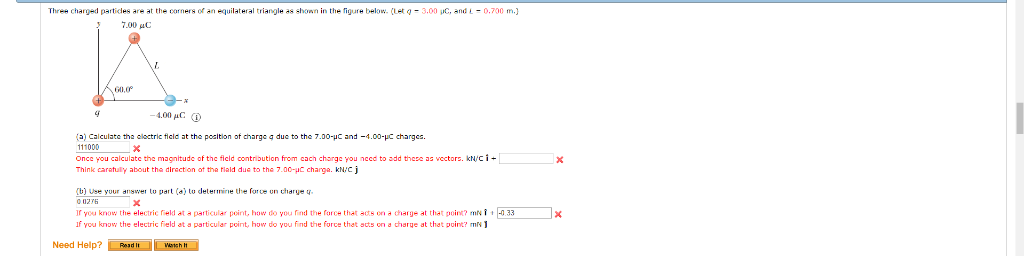Three charged particdes are at the carners of an equilateral triangle as shown in the fiqure below. (Let a 3.00 uC, and 0.700 m. 700 aC G0.0 4.00 pC (a) Calculate the clectric ficld at the pocition of charge a due to the 7.00-uC and -4.00-C charges. d there as vectors, kN/Ci u calculate the magnitude of the ficld contribution from cach charge you need to a the tiald duc to the 7.00-uC charge, kN/c i Think caratuly about the direction a (b) Use vour answer to part (e to delermine the force on charue u o 0276 eSU on a dhatge at thel pon ls .33 acts on a tharge at that point? mN Ir you know the electric rield at a particular point, how do you find f you know the electric fiel a particular Need Help?Read ItWa1eh H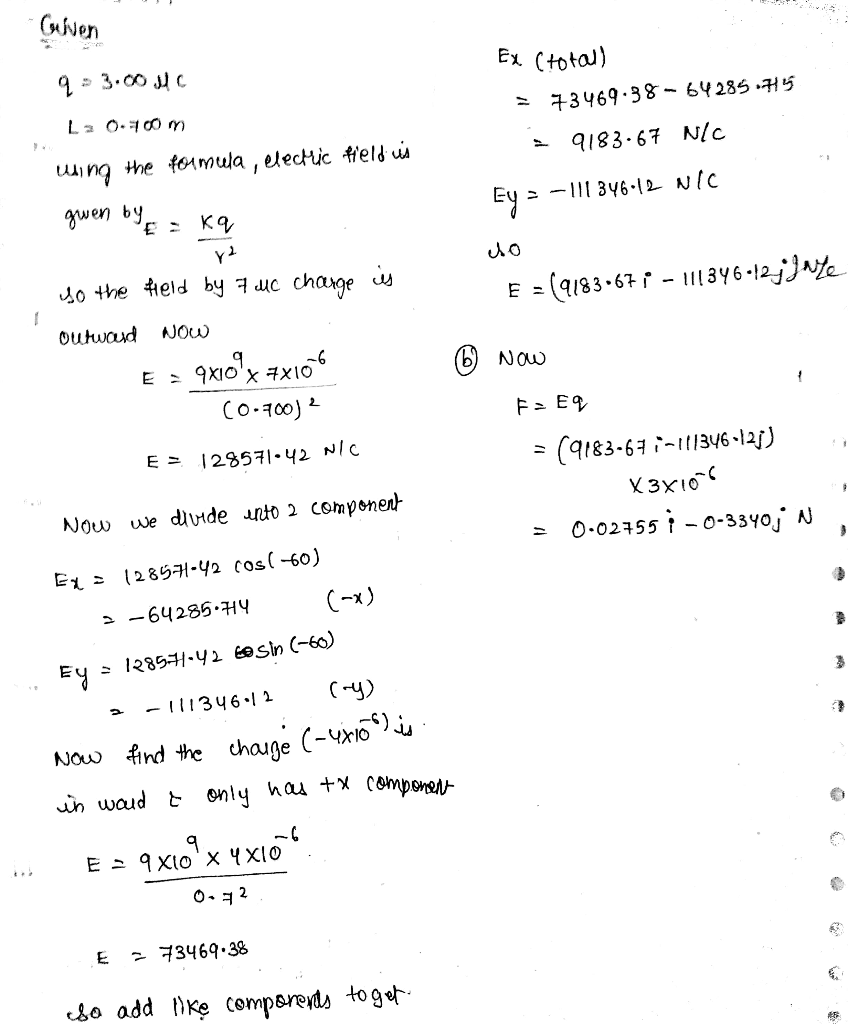#### Earn Coin

Coins can be redeemed for fabulous gifts.

Similar Homework Help Questions
• ### Three charged particles are at the corners of an equilateral triangle as shown in the figure...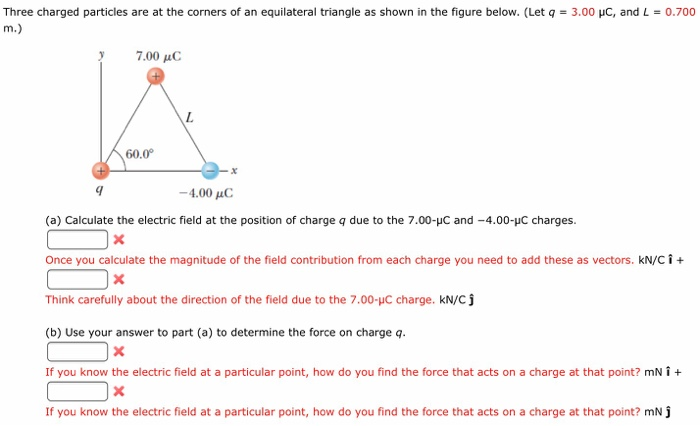Three charged particles are at the corners of an equilateral triangle as shown in the figure below. (Let q = 3.00 pC, and L = 0.700 y 7.00 μC 60.0 -4.00 C (a) Calculate the electric field at the position of charge q due to the 7.00-HC and -4.00-uC charges. Once you calculate the magnitude of the field contribution from each charge you need to add these as vectors. kN/C + Think carefully about the direction of the field due...

• ### Three charged particles are at the corners of an equilateral triangle as shown in the figure...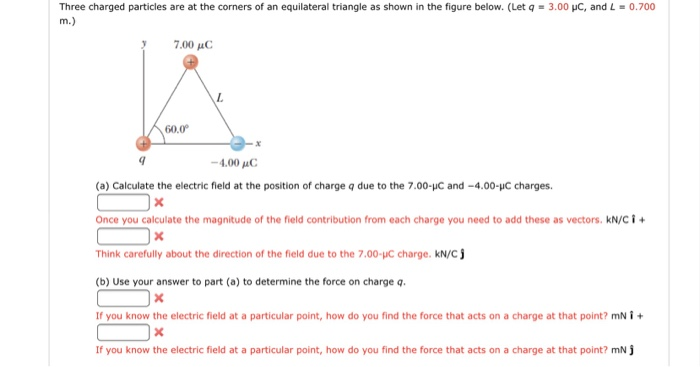Three charged particles are at the corners of an equilateral triangle as shown in the figure below. (Let q = 3.00 pc, and L-0.700 7.00 AC 60.0 -4.00 μC (a) Calculate the electric field at the position of charge q due to the 7.00-C and -4.00-C charges. Once you calculate the magnitude of the field contribution from each charge you need to add these as vectors. kNC Think carefully about the direction of the field due to the 7.00-pC charge....

• ### help!!!! Three charged particles are at the corners of an equilateral triangle as shown in the...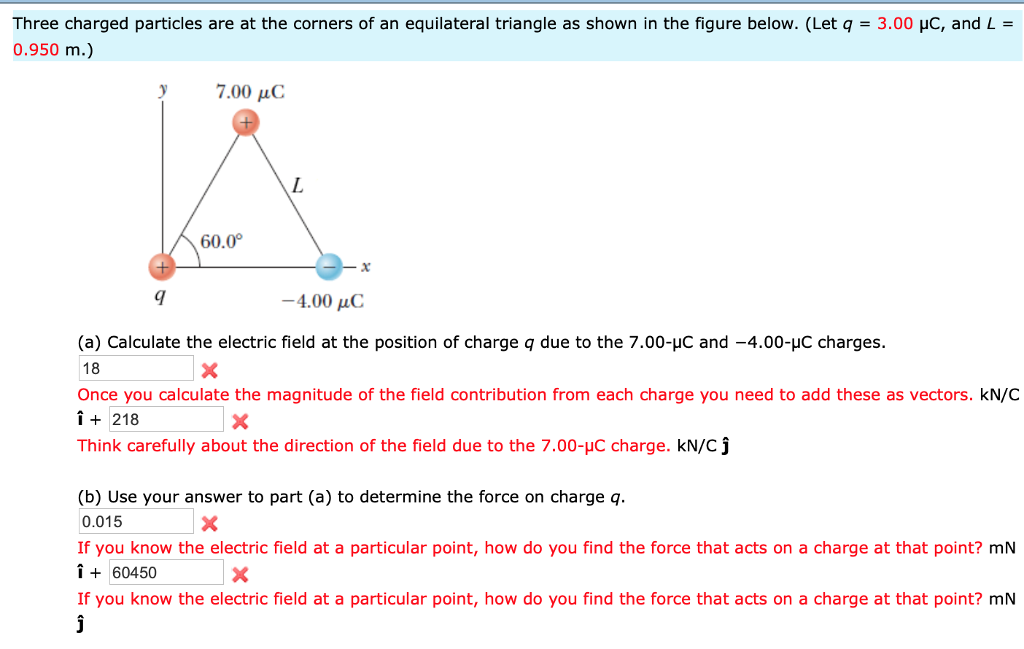help!!!! Three charged particles are at the corners of an equilateral triangle as shown in the figure below. (Let q = 3.00 μC, and L- 0.950 m.) 7.00 μC 60.0° -4.00 μC (a) Calculate the electric field at the position of charge q due to the 7.00-HC and -4.00-HC charges. 18 Once you calculate the magnitude of the field contribution from each charge you need to add these as vectors. kN/C i218 Think carefully about the direction of the field...

• ### Three charged particles are at the corners of an equilateral triangle as shown in the figure...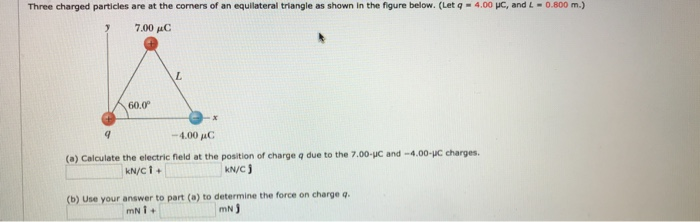Three charged particles are at the corners of an equilateral triangle as shown in the figure below. (Let q-4.00 pe, and 4-0800 m.) 7.00 μο 60.0 -4,00 μο: (o) Calculate the electric fleld at the postion of charge q due to the 7.00-JC and -4.00-uC charges. kN/C j (b) Use your answer to part (a) to determine the force on charge q mN)

• ### Question6 Three charged particles are located at the corners of an equilateral triangle as shown in...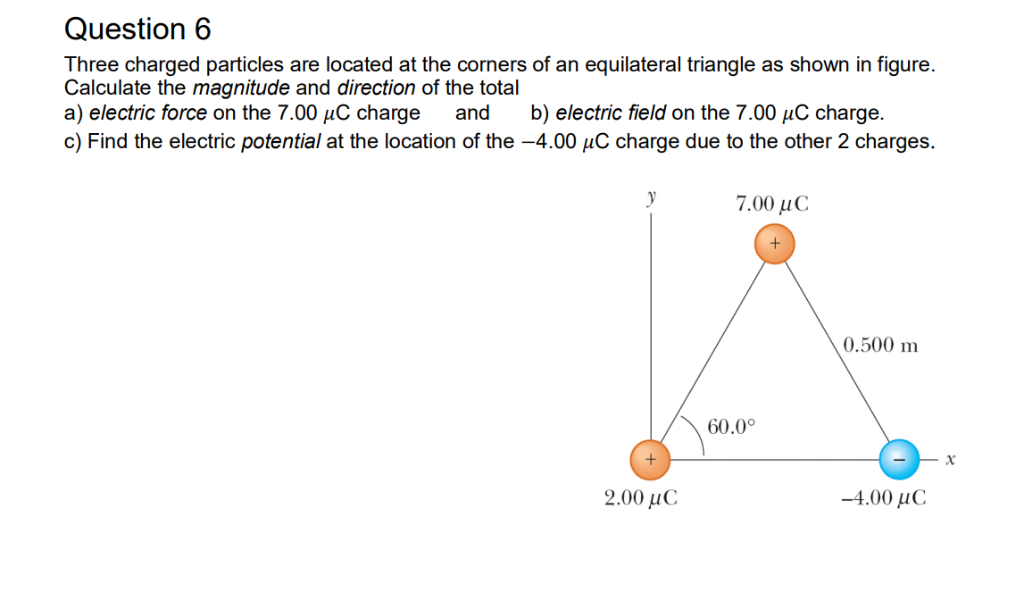Question6 Three charged particles are located at the corners of an equilateral triangle as shown in figure Calculate the magnitude and direction of the total a) electric force on the 7.00 HC charge and b) electric field on the 7.00 uC charge c) Find the electric potential at the location of the-4.00 μC charge due to the other 2 charges. 7.00 μ C 0.500 m 60.0° 2.00 uC -4.00 uC

• ### Three charged particles are at the corners of an equilateral triangle as shown in the figure...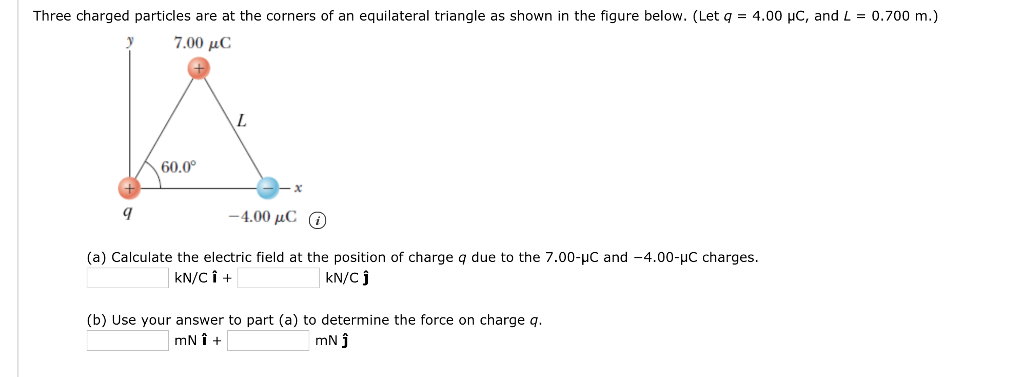Three charged particles are at the corners of an equilateral triangle as shown in the figure below. (Let q = 4.00 yC, and L = 0.700 m.) 7.00 , C 60.0 9 -4.00 pC (a) Calculate the electric field at the position of charge q due to the 7.00-με and-4.00-IC charges. N/Cj (b) Use your answer to part (a) to determine the force on charge q mN

• ### Three charged particles are at the corners of an equilateral triangle as shown in the figure...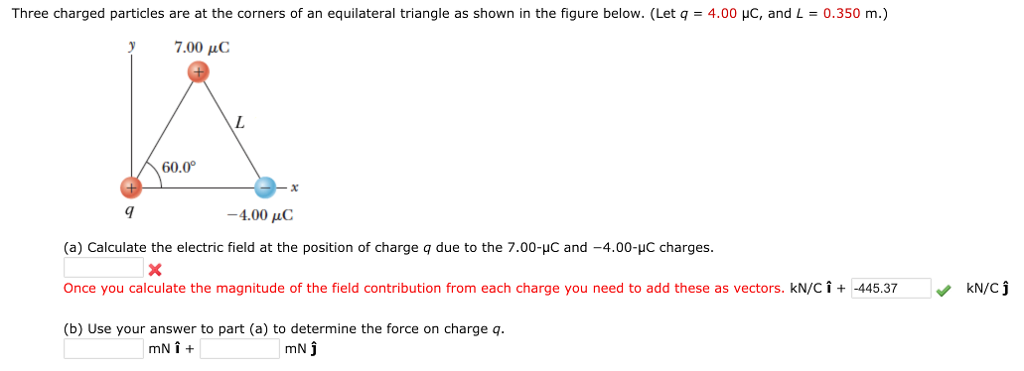Three charged particles are at the corners of an equilateral triangle as shown in the figure below. (Let q = 4.00 μC, and L = 0.350 m.) 7.00 C 60.0 -4.00 pC (a) Calculate the electric field at the position of charge q due to the 7.00-HC and -4.00-HC charges. Once you calculate the magnitude of the field contribution from each charge you need to add these as vectors. kN/C i + -445.37 (b) Use your answer to part (a)...

• ### Three charged particles are located at the corners of an equilateral triangle as shown in the...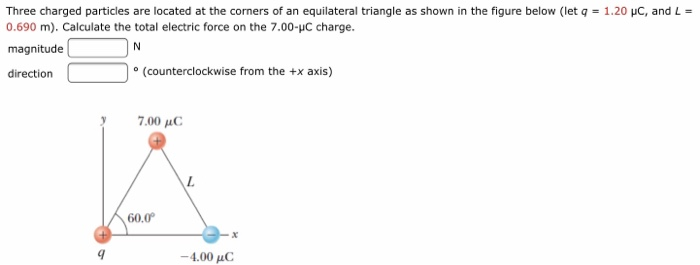Three charged particles are located at the corners of an equilateral triangle as shown in the figure below (let q = 1.20 pC, and L 0.690 m). Calculate the total electric force on the 7.00-uC charge. magnitude direction o (counterclockwise from the +x axis) 7.00 μο 60.0 -4.00 uC

• ### Three charged particles are located at the corners of an equilateral triangle as shown in the...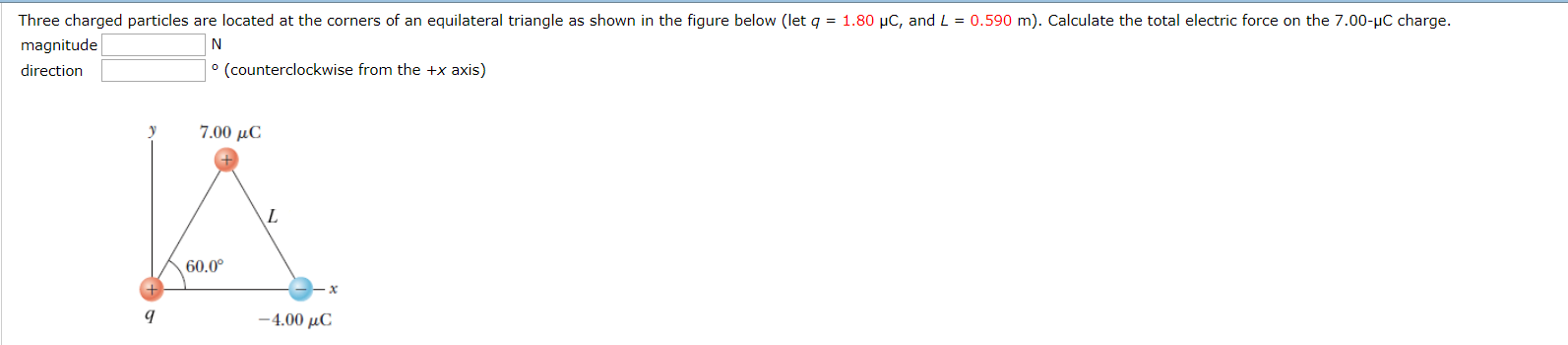Three charged particles are located at the corners of an equilateral triangle as shown in the figure below (let q = 1.80 C, and L = 0.590 m). Calculate the total electric force on the 7.00-C charge. magnitude direction (counterclockwise from the +x axis) 7.00 uc 60.00 - x -4.00 uC

• ### Three charged particles are at the corners of an equilateral triangle as shown in the figure...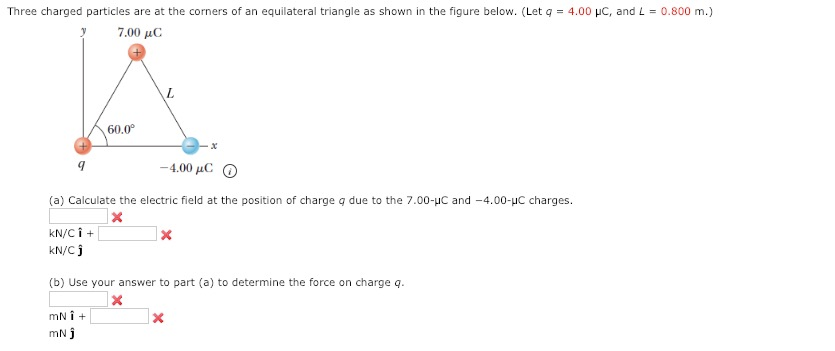Three charged particles are at the corners of an equilateral triangle as shown in the figure below. (Let q 4.00 μC, and L-0.800 m.) 7.00 μο: 60.0° -4.00 pC (a) Calculate the electric field at the position of charge q due to the 7.00-HC and -4.00-HC charges. kN/Ci + kN/Cj (b) Use your answer to part (a) to determine the force on charge q. mN